# Base Converting and Binary Operators

PHP

Share:

#### Free JavaScript Book!Write powerful, clean and maintainable JavaScript.

RRP \$11.95

This article discusses PHP’s binary operators and how to convert between different counting systems. Most programming books and articles only dedicate a page or two to such topics, and although using the operators is really quite simple, there’s a fair amount of background knowledge one must have to use them correctly.

Instead of giving the same bare-bones treatment that every other reference gives, I’ll first provide you the necessary background in number theory. Armed with that knowledge, you’ll be able to understand the binary counting system and base conversions… and familiarity with binary digits is the key to successful use of the binary operators! So without further delay, let’s get started!

## Number Theory

The counting system with which we’re all familiar can be thought of as a series of packages and containers. Each package is worth one unit and is bundled together with other packages and placed in an appropriate container. Each container can only hold a certain amount of units and the units must be packaged into proper packages for that container.

In our Base-10 counting system, units are bound together in multiples of 10. That means we can have up to nine single units, but once a 10th unit is added they all must be packaged together to make a single bundle.There are no surprises there, but the magic lies within the containers. Let’s place a series of buckets side by side and restrict ourselves to filling only the right-most containers. Once one bucket is full, its units are bundled up and moved to the neighboring container. That is, the right-most container can only hold nine single units. A 10th unit would be bundled with the first nine and the whole bundle is moved to the container to its left.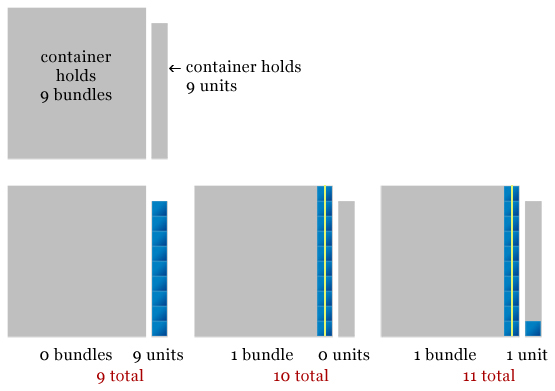Each container can only hold nine of whatever size packages it holds. The right-most container can only hold nine units before they get bundled. The container to its left can only hold 9 bundles. A 10th bundle gets rebundled together and moved the neighboring container. As the containers continue to fill up, the cycle repeats itself; the container to the left can always hold nine bundles of whatever the right container can hold.

The base-10 counting system we’re most familiar with is called decimal, but other counting systems exist. The key is that the limit of each container is one less that of the base. For example, the limit of each container in an octal counting system (base-8) is 7. In hexadecimal (base-16), each container holds 15 units. In hexadecimal, the letters A through F are often used to represent the quantities 10 through 15.

In binary (base-2) each container is limited to one unit or bundle.Since each container is limited to one unit or bundle, a value that makes up a binary number can only be a one or a zero. It is either full (a value of one) or empty (a value of zero). When the string of container values is written out it can resemble a series of checkmarks as if on a list: 1 can be considered a checkmark and 0 is the absence of a check.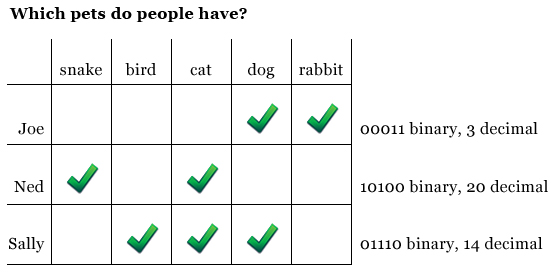## Conversion Functions

Converting values from one base to another can be tedious if done manually depending on the size of the value. To help make life easier, PHP has several built-in functions which can be used for the conversion of numbers from one base to another: `decbin()`, `bindec()`, `decoct()`, `octdec()`, `dechex()`, `hexdec()`, and `base_convert()`.

The `decbin()` function accepts a decimal format number and returns a string representing the binary equivalent. The counterpart function `bindec()` accepts a string representing a binary number and returns the decimal value.

The `decoct()` function accepts a decimal format number and returns a string representing its octal equivalent. Its counterpart `octdec()` accepts the string representation of an octal value and returns the decimal number.

`dechex()` accepts a decimal value and returns a string representation of the hexadecimal value; `hexdec()` accepts a string representing a hexadecimal number and returns the decimal equivalent.

``````<?php
\$num = 21;

echo "Decimal value: \$numn";

echo "Binary value: " . decbin(\$num) . "n";
echo "Octal value: " . decoct(\$num) . "n";
echo "Hexadecimal value: " . dechex(\$num) . "n";``````

In the code above, a decimal value of 21 is stored in the variable `\$num`. The functions `decbin()`, `decoct()`, and `dechex()` are used to display the binary, octal, and hexadecimal equivalents; the binary value is 10101, the octal value is 25, and the hexadecimal value is 15.

The `base_convert()` function is used to convert between arbitrary bases. It accepts two arguments, the first is the original base and the second is the desired base. Let’s assume a particular program needs to convert base-7 and base-11 values; there are no convenience functions to perform these tasks, so `base_convert()` is used.

``````<?php
\$num = 21;

echo "Decimal value: \$numn";

echo "Base-7 value: " . base_convert(\$num, 10, 7) . "n";
echo "Base-11 value: " . base_convert(\$num, 10, 11) . "n";``````

The base-7 value of decimal 21 is 30, and base-11 is 1A.

Granted, base-7 and base-11 certainly seem strange, but they do illustrate how `base_convert()` works. Perhaps more realistically you’ll find yourself using `base_convert()` to convert directly between binary, octal, and hexadecimal values when a convenience function isn’t available.

``````<?php
\$octNum = 25;

echo "Octal value: \$octNumn";
echo "Binary value: " . base_convert(\$octNum, 8, 2) . "n";``````

## Bitwise Operators

Back in the number theory section you saw that each binary container could either be full (have a value of one) or empty (a value of zero). Each package that fills a container we call a “bit”.

### Bitwise-And

The bitwise-and operator is an ampersand (`&`). It accepts two values and logically ands their binary values. Consider the following example: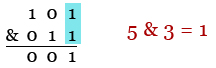The bitwise-and operation takes the binary representation of two numbers and returns a number constructed based on which fields both hav a value of 1. 5 & 3 = 1 since both binary representations only have a 1 value in the right most location.

### Bitwise-Or

The bitwise-or operator is a pipe (`|`). It accepts two values and logically ors their binary values.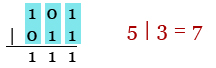The bitwise-or operation takes the binary representation of two number and returns a number constructed based on which fields at least one of have a value of 1. 5 | 3 = 7 since between the two binary representations have a 1 value in each location.

### Bitwise-Exclusive Or (XOR)

The bitwise-xor operator is a caret (`^`). In contrast to the bitwise-or where a 1 is returned for a particular bit so long as at least one of the bits is set, the bitwise-xor will only return one if only one of the bits are set.The bitwise-xor will only return one if only one of the bits are set. If neither of the bits are set, or if both bits are set, zero will be returned. 5 ^ 3 = 6.

### Bitwise Compliment

The compliment operator is a tilde (`~`). It flips the bits in a value so that positions that were 1 would then be 0, and positions that were zero would then be 1.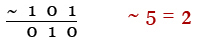Since the compliment switches the values of bits from 1 to 0 and from 0 to 1 in the binary representation of a value, ~5 = 2.

We also briefly saw earlier how binary numbers can be used as a type of checklist; PHP’s bitwise operators can prove helpful in manipulating and masking these tallies by working with each bit.

``````<?php
// people and values from previous chart
\$people = array(
"Joe" => 3,
"Ned" => 20,
"Sally" => 14
);

// mask values if person has certain animal
"rabbit" => bindec("00001"),
"dog" => bindec("00010"),
"cat" => bindec("00100"),
"bird" => bindec("01000"),
"snake" => bindec("10000")
);

foreach (\$people as \$person => \$value) {
// who has a pet dog?
echo "\$person has a dog.n";
}
// who has a snake
echo "\$person has a snake.n";
}
// who does NOT have a cat?
echo "\$person does not have a cat.n";
}
// who has both a cat and a bird?
echo "\$person has both a cat and a bird.n";
}
}``````

The example assigns the data from the pet survey chart you saw earlier into an array with the name \$people. A mask value is then assigned for each animal based on its position in the survey chart. The resulting binary string is converted to a decimal number using `bindec()`. The values are stored in the array \$mask.

A `foreach` loop cycles through each element person and tests for various conditions we’ve set. Each condition uses binary operators to mask out the values we don’t want. If the isolated value matches the value of the mask, it means the survey results matches the criteria we’re looking for. It’s important watch the order of operations though and use parenthesis to ensure the operators work on the correct data.

## Summary

This article definitely covered a lot of material! I provided you with a look at how counting systems are structured, showed you how to convert between various number system, and then how to use binary operators. The article concluded with a simple bit-masking example to pull everything together. Hopefully now you’ll be able to successfully incorporate these seemingly tricky concepts in your scripts with ease!

Image via Fotolia### Timothy Boronczyk

Timothy Boronczyk is a native of Syracuse, New York, where he lives with no wife and no cats. He has a degree in Software Application Programming, is a Zend Certified Engineer, and a Certified Scrum Master. By day, Timothy works as a developer at ShoreGroup, Inc. By night, he freelances as a writer and editor. Timothy enjoys spending what little spare time he has left visiting friends, dabbling with Esperanto, and sleeping with his feet off the end of his bed.

#### New books out now!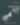Learn how Git works, and how to use it to streamline your workflow!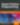Google, Netflix and ILM are Python users. Maybe you should too?